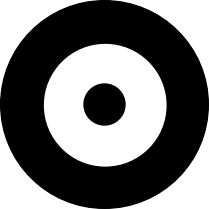# Free SAT Practice Questions

Question 1 of 1
ID: SAT-PSDA-6
Section: Math - Problem Solving & Data Analysis
Topic: Probability
Difficulty level: Challenging

(Practice Mode: Single selected Question » Back to Overview)The above figure shows a circular dartboard. The outer-most black circle is of radius 5 centimeters, the middle white circle is of radius 3 centimeters, and the inner-most black circle is of radius 1 centimeter. If a dart randomly hits the dartboard, what is the probability that it hits any of the black parts of the dartboard? (Calculator is allowed)

A$\frac{1}{3}$
B$\frac{1}{2}$
C$\frac{13}{17}$
D$\frac{17}{25}$